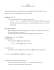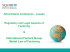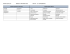# FACTORING ALGEBRAIC EXPRESSIONS Math 7

## Transcription

FACTORING ALGEBRAIC EXPRESSIONS Math 7
```FACTORING ALGEBRAIC
EXPRESSIONS
Math 7
RECALL EXPANDING
EXPRESSIONS
 Before, we used the Distributive Property
to expand an algebraic expression.
 Recall that the two parts of the expression
were called “factors”.
 For example, 3(2x + 9)
 Since the two things are being multiplied together
(using the Distributive Property), the “3” and the
“2x + 9” are said to be factors
NEW TODAY
 Today, we will basically be doing the reverse of
Expanding.
 The reverse of expanding is Factoring.
 Think about the result that you received on the
previous slide when you expanded the
expression 3(2x + 9)….6x + 27
 What if I gave you 6x + 27 and asked you to get
back to the “factored version?” In other words,
get back to what it was prior to expanding it.
FACTORING EXPRESSIONS
 Think about 6x + 27. Look at the two terms 6x and
27.
 What do you notice about both of them?
 Yes…they are both divisible by 3. Thus, we say that
they share a common factor of 3.
 Since this is the case, you can “factor out” the 3 like
this:
 3(
)
Since you factored out the three from both of the terms,
what is left in each term? To find this, simply divide both
terms by 3.
FACTORING CONTINUED
 6x + 27 and 3(2x + 9) are called
equivalent expressions because they are
equal to each other, but they look different.
 It is possible to have many different
equivalent expressions to the same
expression
EQUIVALENT EXPRESSIONS
 For example:
 12x + 24 has quite a few expressions that are
equivalent to it. Can you give me some? Also,
explain why they are equivalent
 12(x + 2) is said to be the “simplest form” of
12x + 24 because the “greatest common factor” or
“GCF” was factored out (12 is the largest factor
that is in both 12 and 24)
EXAMPLES
 Create an equivalent expression to the following by factoring
out the “common term”
 12x + 30
 14 + 21x
 72y – 36
 6p – 45
MORE EXAMPLES
 54y + 15x – 9
 8m – 24
 21p + 7
MAKE CERTAIN YOU ARE CORRECT
 How can we be sure that we have correctly
created an equivalent expression?
 Use the Distributive Property to see if you
get back to the original expression! This is a
great way to “check” that you are correct.
 Go back and see if this works
FACTORING WITH FRACTIONS
 Obviously fractions and mixed numbers will make things
much more difficult.
 It will be more difficult because it is tough to recognize
what they will actually have in “common”
3
1
x2
4
3
 Take a look:
3
1
x2
4
3
 What do they have in common?
FACTORING WITH FRACTIONS AND
MIXED NUMBERS
The first thing we should do is convert all mixed numbers to
improper fractions. It is easier to deal with JUST
FRACTIONS!
3
1
x2
4
3
3
7
would change to
x
4
3
Looking at the two fractions, what do they share?
Would a common denominator help?
This would make it 9 x  28
12
12
FACTORING WITH FRACTIONS AND
MIXED NUMBERS
 Taking a look at
 9
(___  ___)
9
28 you realize that they share….
x
12
12
How do we come up with the inside?
28 9

12 12
12
 Divide, remember? So you must do 9
12
And you must do 28
x
9
x
12
9

12 12
DROP SWITCH FLIP
FACTORING WITH FRACTIONS AND
MIXED NUMBERS
 Thus, the factored version would be:
3
1
(x  3 )
4
9
 In actuality, there are in infinite amount of equivalent
expressions that we could come up with.
 We are essentially free to take out whatever we want,
because we can always divide a fraction away from another
fraction….lets take a look at this concept on the next slide.
FACTORING WITH FRACTIONS AND
MIXED NUMBERS
3
7
x
4
3
FACTORING WITH FRACTIONS AND
MIXED NUMBERS
 On a state test, you are more likely to see these as
multiple choice questions. If this is the case, the
best way to approach them is to just expand all of
the choices out to see which one will get you the
original in return
 Example: Take a look at NYS Testing Program
Sample Question…coming right up
EXAMPLE FROM NYS TEST
 http://www.p12.nysed.gov/assessment/common-core-
 Another Example:
4
1
x  2 is equivalent to which expression below?
5
4
A) 4(5x + 2)
B) 1 (16 x  8)
20
C)
2
5
(2 x  5 )
5
8
```

### 1. Make high quality graphs of the following by hand. Label the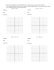### CH 4.4 Factoring Quadratic Expressions GOAL: Factor quadratic expressions REVIEW: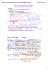### ShadowCon Talk (Graham Fletcher)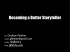### 6.4 Perfect-Square Trinomials and Differences of Squares 2 = ( +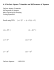### 5.2.2 Solve by Factoring - Social Circle City Schools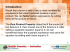###  I know how to identify polynomials and name them by... degrees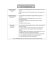### Sample Pages - The Mailbox### www.patha.org### www.highlane.stockport.sch.uk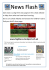### Factoring Using the Distributive Property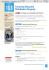### TSIA Test Prep Luis Molina John Cox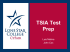### 1 TI-30X II CALCULATOR OPERATIONS When using the calculator